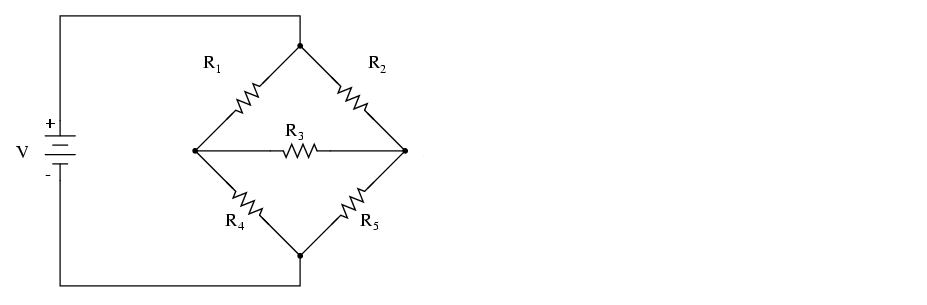# Problem: In the following circuit, the resistances are R 1 = 5 Ω, R2 = 10 Ω, R3 = 10 Ω, R4 = 25 Ω, and R5 = 15 Ω. If the current through R3 is 1 A to the right, what is the voltage of the battery?

###### FREE Expert Solution
85% (304 ratings)
###### Problem Details

In the following circuit, the resistances are R 1 = 5 Ω, R2 = 10 Ω, R3 = 10 Ω, R4 = 25 Ω, and R5 = 15 Ω. If the current through R3 is 1 A to the right, what is the voltage of the battery?